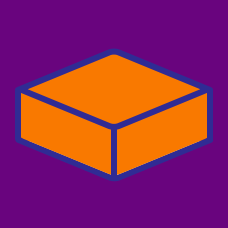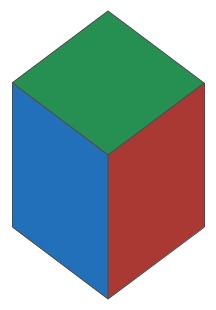Geometry

# Surface Area - Problem SolvingThe volume of a cube with positive side length is numerically equal to the surface area of a cube (though they will have different units). What is the volume of the cube?

The surface area of a sphere of radius 1 is equal to the surface area of a cylinder with a circular base of radius 1. What is the height of the cylinder?

The volume of a sphere is numerically equal to the surface area of the sphere. If this value is equal to $N \pi$, what is the value of $N$?

Brilli the ant is playing with a ball of radius $R$, which is a positive integer. Given that the surface area and volume of the ball are $N\pi$ and $M\pi$, where $N$ and $M$ are 4-digit integers, what is $R$?

Details and assumptions

The number $12=012$ is a 2-digit number, not a 3-digit number.

If the surface area of a sphere is numerically equal to the volume of the sphere, what is the radius?

×